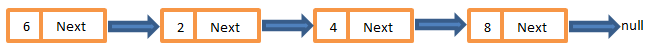# Introduction to Linked List Data Structure and Analysis on Search, Insert and Delete time

 Category: Data Structure And Algorithms Tags:Linkedlist Code Files

## Introduction

Linked List is a data structure where objects are arranged in a linear order, unlike array which uses continuous memory locations instead it uses pointers where locations might be dynamic(not continuous) and every node must be having a pointer called “next” which contains next element’s location. If pointer “next” is null means linked list is ended and no more elements are connected further.

A linked list has connected nodes, a node contains two properties value and next, where value is node's value and next is pointer which connects a node to further node. Where a doubly linked list is same but has two pointers one is next and other is previous, next is same in this case but previous pointer is to point predecessor of current node, so for first node(head) of list pointer "previous" will be null and for last node pointer "next" will be null.Fig 1: A Singly Linked List Example

1. When user needs inexpensive insertion/deletion because at any point user can insert/delete the nodes
2. When you are unpredictable about the number of elements(size of list) because in linked list you no need to declare the size
3. No need to move elements when you insert/delete

## Implementation

Below we are going to implement doubly linked List, for that we create structure of Node:

```public class Node
{
public int Value { get; set; }
public Node Next { get; set; }
public Node Previous { get; set; }
}
```

Above class is having one value and two pointers as we read in introduction, Value shows the value of node, Next & Previous are pointers to point next and previous nodes respectively, now we will create a class which actually connects the series of nodes we call it DoublyLinkedList:

```public class DoublyLinkedList
{
public Node Tail;
{
}
{
}
public void InsertAtTail(int element)
{
}    public void InsertAtPosition(int element, int position)    {    }
public bool Delete(int value)
{
}
public Node Search(int value)
{
}
}
```

Above I have given you structure of class having members and methods we are going to implement, this class contains two pointers Head, Tail and four methods which are InsertAtHead, InsertAtTail, InsertAtPosition, Delete, Search. Head is starting point of list and tail is end point. Below are implementation of each of method:

```public void InsertAtHead(int element)
{
{
}
else
{
Node newNode = new Node();
newNode.Value = element;
}
}
```

Above method first checks if head null, if it is then it assigns value directly to head and head and tail are equal because only one element is present in list, if head isn't null then it creates a new element assigns value to it and then in next pointer of new element it sets current Head and finally makes new node as Head by assigning new node to Head.

InsertAtTail:

```public void InsertAtTail(int element)
{
{
}
else
{
Node newNode = new Node();
newNode.Value = element;
Tail.Next = newNode;
newNode.Previous = Tail;
Tail = newNode;
}
}
```

InsertAtPosition

`public void InsertAtPosition(int element, int position){    if (Head == null)    {        Head = new Node();        Head.Value = element;        Tail = Head;    }    else    {        Node newNode = new Node();        newNode.Value = element;        Node temp = Head;        while(temp != null && position > 1)        {            temp = temp.Next;            position--;        }        Node tempPre = temp.Previous;        tempPre.Next = newNode;        newNode.Previous = tempPre;        newNode.Next = temp;        temp.Previous = newNode;    }}`

Above method reaches at given position using loop and inserts new element at that position.

Delete:

```public bool Delete(int value)
{
while (node != null)
{
if (node.Value == value)
{
node.Previous.Next = node.Next;
node.Next.Previous = node.Previous;
return true;
}
else
node = node.Next;
}
return false;
}
```

Above method iterates the loop and search for the node which value is equal to input value, if finds it, then it makes next pointer of predecessor directly to current node's successor and successor's previous pointer to current node's predecessor. It is something like skipping the current node in series.

Search:

```public Node Search(int value)
{
while (node != null)
{
if (node.Value == value)
return node;
else
node = node.Next;
}
return null;
}
```

Search is again same thing looking for the value in every node. Below I'm going to execute the this code like:

```DoublyLinkedList list = new DoublyLinkedList();list.InsertAtHead(5);list.InsertAtHead(15);list.InsertAtTail(20);list.InsertAtTail(25);list.InsertAtPosition(4, 3);Console.WriteLine("After Inserting all");Node node = list.Head;//this is traversing the listwhile (node != null){    Console.WriteLine(node.Value);    node = node.Next;}//after deletevar del = list.Delete(20);var ser = list.Search(25);node = list.Head;Console.WriteLine("After Deleting 20");//this is traversing the listwhile (node != null){    Console.WriteLine(node.Value);    node = node.Next;}Console.ReadLine();
```

In above code, using while loop we can see how to traverse the list and print all the node values. The output will be:

After Inserting all
15
5
4
20
25
After Deleting 20
15
5
4
25

## Analysis

As we seen above insertion at Head/Tail can be done in O(1) time that is quit fast if your requirement is to insert at ends. But insertion in middle like Insertion at some position then you will have to traverse the list until you find the position and then you have to insert new node so that will be O(n+1) time expensive operation in worst cast.

Deletion and search complexity is also O(n) in worst case, because it has to traverse the list to find the correct node, traversing is always O(n) where n is the number of nodes in list.

 Like0 PeopleNikhil Joshi Ceo & Founder at Dotnetlovers Atricles: 146 Questions: 16 Given Best Solutions: 16 *

Login via:x